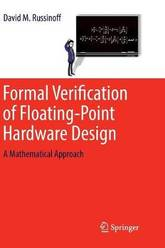Filter
(found 31 products)This is the first book to focus on the problem of ensuring the correctness of floating-point hardware designs through mathematical methods. Formal Verification of Floating-Point Hardware Design advances a verification methodology based on a unified theory of register-transfer logic and floating-point arithmetic that has been developed and applied to the ...
Formal Verification of Floating-Point Hardware Design: A Mathematical Approach
This is the first book to focus on the problem of ensuring the correctness of floating-point hardware designs through mathematical methods. Formal Verification of Floating-Point Hardware Design advances a verification methodology based on a unified theory of register-transfer logic and floating-point arithmetic that has been developed and applied to the formal verification of commercial floating-point units over the course of more than two decades, during which the author was employed by several major microprocessor design companies. The book consists of five parts, the first two of which present a rigorous exposition of the general theory based on the first principles of arithmetic. Part I covers bit vectors and the bit manipulation primitives, integer and fixed-point encodings, and bit-wise logical operations. Part II addresses the properties of floating-point numbers, the formats in which they are encoded as bit vectors, and the various modes of floating-point rounding. In Part III, the theory is extended to the analysis of several algorithms and optimization techniques that are commonly used in commercial implementations of elementary arithmetic operations. As a basis for the formal verification of such implementations, Part IV contains high-level specifications of correctness of the basic arithmetic instructions of several major industry-standard floating-point architectures, including all details pertaining to the handling of exceptional conditions. Part V illustrates the methodology, applying the preceding theory to the comprehensive verification of a state-of-the-art commercial floating-point unit. All of these results have been formalized in the logic of the ACL2 theorem prover and mechanically checked to ensure their correctness. They are presented here, however, in simple conventional mathematical notation. The book presupposes no familiarity with ACL2, logic design, or any mathematics beyond basic high school algebra. It will be of interest to verification engineers as well as arithmetic circuit designers who appreciate the value of a rigorous approach to their art, and is suitable as a graduate text in computer arithmetic.
104.990000 USD

## Formal Verification of Floating-Point Hardware Design: A Mathematical Approach

by David M. Russinoff
Paperback / softbackSoftware Engineering Education: 7th SEI Csee Conference, San Antonio, Texas, USA, January 5-7, 1994. Proceedings
USD

## Software Engineering Education: 7th SEI Csee Conference, San Antonio, Texas, USA, January 5-7, 1994. Proceedings

by SEI Conference on Software Engineering Education, Jorge L. Diaz-Herrera
Paperback / softback
Page 1 of 2